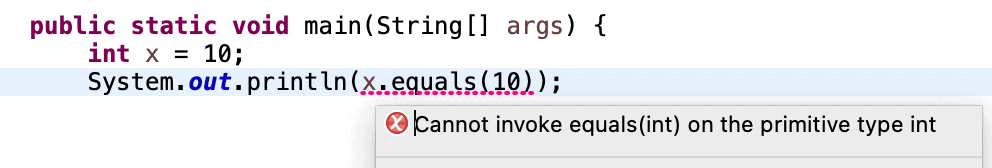## 1. Overview

In this tutorial, we’ll take a closer look at the Java error, “int cannot be dereferenced”. First, we’ll create an example of how to produce it. Next, we’ll explain the leading cause of the exception. And finally, we’ll see how to fix it.

## 2. Practical Example

Now, let’s see an example that generates a compilation error, “X cannot be dereferenced”.

Here, X represents one of the eight Java primitives, namely intbyteshortlongfloatdoubleboolean, and char.

First, let’s create a class Test and compare an int to some other value:

``````int x = 10;
System.out.println(x.equals(10));``````

When compiling the code from the terminal, we’ll get the error:

``````\$ javac Test.java
Test.java:8: error: int cannot be dereferenced
System.out.println(x.toString());
^
1 error``````

Also, modern IDEs like Eclipse and IntelliJ will show an error without even compiling:## 3. Cause

In Java, a reference is an address to some object/variable. Dereferencing means the action of accessing an object’s features through a reference. Performing any dereferencing on a primitive will result in the error “X cannot be dereferenced”, where X is a primitive type. The reason for this is that primitives are not considered objects — they represent raw values:

``````int x = 10;
System.out.println(x.equals(10));``````

When building the code from the terminal, we’ll get the error “int cannot be dereferenced”.

However, with Object, it works fine:

``````Object testObj = new Object();
testObj.toString();``````

Here, testObj is an object, and dereferencing happens on calling toString() with the . operator on testObj. This will not give any error as testObj is an object and, thus, dereferencing will work.

## 4. Solution

In our example, we need to check the equality of the two values.

The first solution to our problem is to use == instead of equals() for primitive types:

``````int x = 10;
System.out.println(x == 10);``````

When we run the code, it will print “true”.

The second solution is to change the primitive to a wrapper class.

Java provides wrapper class objects for each primitive type.

For instance, we can convert primitive types to a wrapper object if we must use equals():

``````Integer x = 10;
System.out.println(x.equals(10));``````

This error does not have a one-size-fits-all solution. Depending on the use case, we may use either of the above two solutions.

## 5. Conclusion

We’ve explained Java’s “int cannot be dereferenced” error. Then, we discussed how to produce the error and the cause of the exception. Lastly, we discussed a solution to resolve the error.

### Get started with Spring 5 and Spring Boot 2, through the Learn Spring course:

>> CHECK OUT THE COURSE